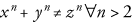## equation

equation — A displayed mathematical equation.

equation ::=

## Attributes

• floatstyle
• label
• pgwide (enumeration)
• “0”
• “1”
• type

• example must not occur among the children or descendants of equation
• figure must not occur among the children or descendants of equation
• table must not occur among the children or descendants of equation
• equation must not occur among the children or descendants of equation
• If this element is the root element, it must have a version attribute.

## Description

An `equation` is a formal mathematical equation (with an optional rather than a required title).

If the MathML Module is used, `equation` can also contain the mml:math element.

### Processing expectations

Formatted as a displayed block. For an inline equation, use `inlineequation`.

Processing systems that number equations or build a table of equations at the beginning of a document may have difficulty correctly formatting documents that contain both `equation`s with `title`s and `equation`s without `title`s. You are advised to use `informalequation` for equations without titles.

### Attributes

floatstyle

Specifies style information to be used when rendering the float

label

Specifies an identifying string for presentation purposes

pgwide

Indicates if the element is rendered across the column or the page

type

Identifies the type of equation

### Children

The following elements occur in `equation`: `alt`, `caption` (db.caption), `info` (db.titleforbidden.info), `info` (db.titleonly.info), `mathphrase`, `mediaobject`, `mml:*`, `title`, `titleabbrev`.

## Examples

```1`<article xmlns='http://docbook.org/ns/docbook'>`
`<title>Example equation</title>`
` `
`<equation xml:id="eq.fermat">`
5`<title>Fermat's Last Theorem</title>`
`  <alt>x^n + y^n ≠ z^n ∀ n ≠ 2</alt>`
`  <mediaobject>`
`    <imageobject condition="print">`
`      <imagedata fileref="figs/print/db5d_refeqn01.pdf"/>`
10`    </imageobject>`
`    <imageobject condition="web">`
`      <imagedata fileref="figs/web/db5d_refeqn01.png"/>`
`    </imageobject>`
`  </mediaobject>`
15`</equation>`
` `
`</article>`
```Equation 1.1 Fermat's Last Theorem

Alternatively, for relatively simple equations such as Fermat's Last Theorem, `mathphrase` is often sufficient:

```1`<article xmlns='http://docbook.org/ns/docbook'>`
`<title>Example equation</title>`
` `
`<equation xml:id="eq.fermat.mathphrase">`
5`<title>Fermat's Last Theorem</title>`
`  <alt>x^n + y^n ≠ z^n ∀ n ≠ 2</alt>`
`  <mathphrase>x<superscript>n</superscript>`
`+ y<superscript>n</superscript>`
`≠ z<superscript>n</superscript>`
10`∀ n ≠ 2</mathphrase>`
`</equation>`
` `
`</article>`
```
xn + yn ≠ zn ∀ n ≠ 2
Equation 2.1 Fermat's Last Theorem